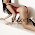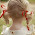# geeky.blogger

## Human Motion Animation with Inverse Kinematics

Friday, April 25, 2008 Posted by Ismail Habib

Human body consists of at least a hundred joint that are connected in some way. When we try to move our hand, there are at least three joint that moves (i.e. shoulder, elbow and wrist joint). Some of human body joints are also capable in moving in 3 dimensions (e.g. shoulder, hip and neck joint). Off course, as a human, we do our movement intuitively, without trying to calculate how can we reach a position (e.g. walking, reaching, looking around, etc). But, in order to animate a human movement using a computer, we have to specify the angle of rotation for each joint in order to perform a movement. The basic procedure to animate a human body movement is to use kinematics. Kinematics is one of the fields of mechanics that evaluates the position changes of an object in a period of time without considering masses or forces of the object itself. The easiest way to apply kinematics in human animation is by using a so-called forward kinematics. Forward kinematics is a low level technique for animating an articulated structure. Using this method, the animator has a great degree of freedom to control the animation, because animator has to define the angle for each body joint. However, it might take a lot of effort to define all the components of the animation for only a single movement.

On the other hand, there is inverse kinematics which could be classified as a high level approach of animating an articulated structure. Instead of defining every transformation of joints, the animator can just define the destination point of end part of the articulated structure (i.e. hand or feet), and use that position to calculate the transformation for each joint, in backward fashion (i.e. calculate the wrist joint transformation first then elbow joint and shoulder joint as the last one).

Using inverse kinematics, it is possible to get several solutions or nothing at all. The drawback of this method is that the complexity of a structure might be very high that would lead to a condition where the calculation becomes too expensive or even impossible. The inverse kinematic could pose a non-linear problem where given a certain position x, there could be multiple solution in order to reach that position.

Inverse Kinematics

By knowing the starting and the ending position of the end-effectors (i.e. hand or feet), we then try to calculate the movement of the whole articulated structure (e.g. hand movement or walking movement). The basic idea of inverse kinematic is to find the appropriate angle of every joint in the articulated structure, given a desired position. Mathematically, inverse kinematics could be expressed as:

Θ = f-1 (x) (1)

Where Θ is the joint angle and x is the end-effector position. Since articulated structures consist of several joints, the equation above would result in a non-linear equation. So for a given end-effector location x, there might be more than one solution for Θ. For solving inverse kinematics, there are three main methods that could be used, which are:

a) Analytical method The most common way to do analytical method is by creating a closed-form equation that is obtained by trigonometric approach. The problem we faced with this method is the difficulty to obtain the trigonometric equation for every joint that are available.

b) Numerical Method We can solve non-linear equation (1) by transforming it into linear equation (linearization). After linearization, the connection between end-effector and joint angles is expressed with the following equation:

dX/dt = J(Θ) . dΘ/dt (2)

where dX/dt is the end-effector velocity, dΘ/dt is the joint velocity, and J relates the changes in the joint variables with the changes in the position of the end-effector in an M x N manner, where M is the joint variables count and N is the dimensions of the end-effector vector.

J = dF(Θ)/dΘ (3)

Where F is total function matrix for the movement of every joint angle.

Inverting equation (2) will result in:

d Θ /dt = J-1(Θ) . dx/dt (4)

And to solve it is by using iterative algorithm. When dealing with this method, we were faced with a very difficult equation in the Jacobian matrix that is hard to solve even using iterative algorithm because of the complexity of the equation.

c) Optimization Method For this method, what we do is try to solve each joint angle by trying to optimize the value of it. One of the examples of Optimization Method is the Cyclic Coordinate Descent Method (CCD) where we try to improve the angle of each joint, step by step, until the articulated structure could reach the desired end location for end-effector.

The next post will cover more about Cyclic Coordinate Descent Method.

### Related post

1.Anonymous1:28 PM

ngomong apa loe bib? gak ngerti gw..

2.Sama gak ngertinya waktu aku baca blogmu :P

3.Anonymous2:10 AM

berarti kita sama2 ngaco hahahhaha

4.すごい!! ぜんぜん わかりません xD

5.6.7.8.9.10.11.12.13.14.15.16.17.18.19.20.21.22.23.24.25.26.Female Escorts: Mordecai and Hegai ready Esther to be a better queen then Vashti (one:19), whereas all the opposite girls, from the full kingdom, were instructed to proudly show their unique and strange manners and customs to the king.
Female Escorts,High Class Call Girls,Escorts Girls,Escorts ,Escorts near me
Female Escorts

27.I was looking for something like this…I found it quiet interesting, hopefully, you will keep posting such blogs….Keep sharing .
run3.me

28.29.30.شركة تنظيف بالاحساء شركة تنظيف بالاحساء

شركة تنظيف شقق بالدمام شركة تنظيف شقق بالدمام
شركة تنظيف شقق بالمدينة المنورة شركة تنظيف شقق بالمدينة المنورة
شركة تنظيف شقق بالرياض شركة تنظيف شقق بالرياض

31.I accidentally saw your post and it attracted me from the first few seconds, it was excellent, hope you have more successful posts. I do not feel sorry for taking the time to read this post, it is really nice and useful to me, thanks for posting it.
abcya5, friv for school for kids 2019, cá koi mini, io jogos for kids